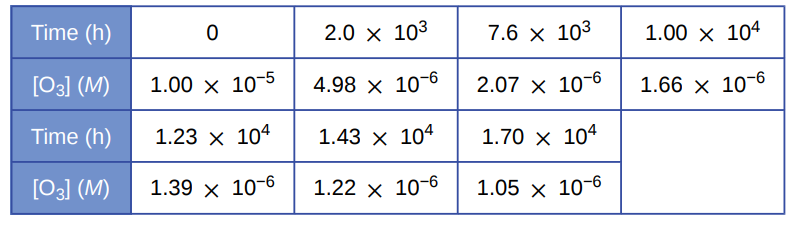# Problem: Pure ozone decomposes slowly to oxygen, 2O3 (g) ⟶ 3O2 (g). Use the data provided in a graphical method and determine the order and rate constant of the reaction.

###### FREE Expert Solution

plot ln[O3] vs t

y = -1.276 x + (-11.8464)

R2 = 0.9272

plot 1/[O3] vs t

y = 50.21 x + 100613.1233

R2 = 0.99999  linear plot

83% (345 ratings)###### Problem Details

Pure ozone decomposes slowly to oxygen, 2O3 (g) ⟶ 3O2 (g). Use the data provided in a graphical method and determine the order and rate constant of the reaction.Frequently Asked Questions

What scientific concept do you need to know in order to solve this problem?

Our tutors have indicated that to solve this problem you will need to apply the Identifying Reaction Order concept. If you need more Identifying Reaction Order practice, you can also practice Identifying Reaction Order practice problems.

What professor is this problem relevant for?

Based on our data, we think this problem is relevant for Professor Windman's class at MC MARICOPA.

What textbook is this problem found in?

Our data indicates that this problem or a close variation was asked in Chemistry - OpenStax 2015th Edition. You can also practice Chemistry - OpenStax 2015th Edition practice problems.